# Natural numbers + arithmetic progression - math problems

#### Number of problems found: 36

• Recurrence relation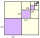Consider a recurrence relation an = 3an-1 - 3an-2 for n = 1,2,3,4,… with initial conditions a1 = 4 and a2 = 2. Calculate a5.
• Sequence 13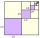2, 2, 3, 5, 9, 11, 17, 21 If the number 23 is added to the list, which measurement will NOT change?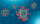A Street has 13 houses in a row. Some resident sin the first house tested positive for Covid-19. The virus spreads in 2 ways: It can spread to the next house or jump directly to the third house. Residents of house 2 can get infected in only one way, house
• Special sequence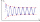What if            2×9=1            3×9=2            4×9=3            5×9=4            6×9=5            7×9=6            8×9=7            9×9=8           10×9=9    then 1×9=??? answer with solutions. .. ..
• Harry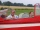Harry Thomson bought a large land in the shape of a rectangle with a circumference of 90 meters. He divided it into three rectangular plots. The shorter side has all three plots of equal length, their longer sides are three consecutive natural numbers. Fi
• The town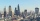The town population is 56000. It is decreasing by 2% every year. What will be the population of the town after 13 years?
• CalculateCalculate the sum of all three-digit natural numbers divisible by five.
• Squirrels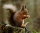The squirrels discovered a bush with hazelnuts. The first squirrel plucked one nut, the second squirrel two nuts, the third squirrel three nuts. Each new squirrel always tore one nut more than the previous squirrel. When they plucked all the nuts from the
• Sum 1-6Find the sum of the geometric progression 3, 15, 75,… to six terms.
• Negative difference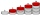Find four arithmetic progression members between 7 and -6.
• Find the meanFind the number between 13 and 29.
• Sum of odd numbersFind the sum of all odd integers from 13 to 781.
• AP membersWhat is the value of x2, x3, x4, x5…of the terms (of arithmetic progression) when x1 = 8 and x6 = 20?
• What areWhat are the four terms between 4 and 19?
• 10th termWhat is the 10th term of the Arithmetic Progression if x1=4 and d=5?
• 1 pageOne page is torn from the book. The sum of the page numbers of all the remaining pages is 15,000. What numbers did the pages have on the page that was torn from the book?
• Finite arithmetic sequenceHow many numbers should be inserted between the numbers 1 and 25 so that all numbers create a finite arithmetic sequence and that the sum of all members of this group is 117?
• Sum of the seventeen numbersThe sum of the 17 different natural numbers is 154. Determine the sum of the two largest ones.
• AM of three numbersThe number 2010 can be written as the sum of 3 consecutive natural numbers. Determine the arithmetic mean of these numbers.
• The sumThe sum of the first 10 members of the arithmetic sequence is 120. What will be the sum if the difference is reduced by 2?

Do you have an exciting math question or word problem that you can't solve? Ask a question or post a math problem, and we can try to solve it.

We will send a solution to your e-mail address. Solved examples are also published here. Please enter the e-mail correctly and check whether you don't have a full mailbox.

Natural numbers - math problems. Arithmetic progression - math problems.